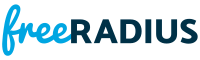`#include <freeradius-devel/radclient.h>`
`#include <freeradius-devel/conf.h>`
`#include <ctype.h>`
`#include <assert.h>`
`#include "smbdes.h"`
`#include "mschap.h"`Include dependency graph for radclient.c:

Go to the source code of this file.

## Typedefs

typedef struct REQUEST REQUEST

## Functions

static int _rc_request_free (rc_request_t *request)

static void deallocate_id (rc_request_t *request)

static int filename_cmp (void const *one, void const *two)

static int filename_walk (UNUSED void *context, void *data)

static int getport (char const *name)

int main (int argc, char **argv)

static void radclient_get_port (PW_CODE type, uint16_t *port)

static int radclient_init (TALLOC_CTX *ctx, rc_file_pair_t *files)

static int recv_one_packet (int wait_time)

static int send_one_packet (rc_request_t *request)

static void NEVER_RETURNS usage (void)

## Variables

static uint16_t client_port = 0

static bool do_output = true

static bool done = true

static rbtree_tfilename_tree = NULL

static int ipproto = IPPROTO_UDP

static int last_used_id = -1

static int packet_code = PW_CODE_UNDEFINED

static fr_packet_list_tpl = NULL

static bool print_filename = false

static char const * proto = NULL

static rc_request_trc_request_tail = NULL

static int resend_count = 1

static int retries = 3

static char const * secret = NULL

static uint16_t server_port = 0

static int sleep_time = -1

static int sockfd

static rc_stats_t stats

static float timeout = 5

## Typedef Documentation

 typedef struct REQUEST REQUEST

Definition at line 37 of file radclient.c.

## Function Documentation

 static int _rc_request_free ( rc_request_t * request )
static

Definition at line 117 of file radclient.c.Here is the caller graph for this function:

 static bool already_hex ( VALUE_PAIR * vp )
static

Definition at line 255 of file radclient.c.Here is the caller graph for this function:

 static void deallocate_id ( rc_request_t * request )
static

Definition at line 787 of file radclient.c.Here is the call graph for this function:Here is the caller graph for this function:

 static int filename_cmp ( void const * one, void const * two )
static

Definition at line 758 of file radclient.c.Here is the caller graph for this function:

 static int filename_walk ( UNUSED void * context, void * data )
static

Definition at line 771 of file radclient.c.Here is the call graph for this function:Here is the caller graph for this function:

 static int getport ( char const * name )
static

Definition at line 187 of file radclient.c.Here is the caller graph for this function:

 int main ( int argc, char ** argv )

Definition at line 1141 of file radclient.c.Here is the call graph for this function:

 static int mschapv1_encode ( RADIUS_PACKET * packet, VALUE_PAIR ** request, char const * password )
static

Definition at line 143 of file radclient.c.Here is the call graph for this function:Here is the caller graph for this function:

 static PW_CODE radclient_get_code ( uint16_t port )
static

Definition at line 233 of file radclient.c.Here is the call graph for this function:Here is the caller graph for this function:

 static void radclient_get_port ( PW_CODE type, uint16_t * port )
static

Definition at line 200 of file radclient.c.Here is the call graph for this function:Here is the caller graph for this function:

 static int radclient_init ( TALLOC_CTX * ctx, rc_file_pair_t * files )
static

Definition at line 282 of file radclient.c.Here is the call graph for this function:Here is the caller graph for this function:

 static int radclient_sane ( rc_request_t * request )
static

Definition at line 728 of file radclient.c.Here is the caller graph for this function:

 static int recv_one_packet ( int wait_time )
static

Definition at line 976 of file radclient.c.Here is the call graph for this function:Here is the caller graph for this function:

 static int send_one_packet ( rc_request_t * request )
static

Definition at line 811 of file radclient.c.Here is the call graph for this function:Here is the caller graph for this function:

 static void NEVER_RETURNS usage ( void )
static

Definition at line 81 of file radclient.c.Here is the caller graph for this function:

## Variable Documentation

static

Definition at line 56 of file radclient.c.

 uint16_t client_port = 0
static

Definition at line 57 of file radclient.c.

 bool do_output = true
static

Definition at line 45 of file radclient.c.

 bool done = true
static

Definition at line 53 of file radclient.c.

 rbtree_t* filename_tree = NULL
static

Definition at line 67 of file radclient.c.

 int ipproto = IPPROTO_UDP
static

Definition at line 65 of file radclient.c.

 int last_used_id = -1
static

Definition at line 60 of file radclient.c.

 int packet_code = PW_CODE_UNDEFINED
static

Definition at line 50 of file radclient.c.

 fr_packet_list_t* pl = NULL
static

Definition at line 68 of file radclient.c.

 bool print_filename = false
static

Definition at line 54 of file radclient.c.

 char const* proto = NULL
static

Definition at line 63 of file radclient.c.

static
Initial value:
", built on " __DATE__ " at " __TIME__

Definition at line 75 of file radclient.c.

 rc_request_t* rc_request_tail = NULL
static

Definition at line 73 of file radclient.c.

static

Definition at line 72 of file radclient.c.

 int resend_count = 1
static

Definition at line 52 of file radclient.c.

 int retries = 3
static

Definition at line 42 of file radclient.c.

 char const* secret = NULL
static

Definition at line 44 of file radclient.c.

static

Definition at line 51 of file radclient.c.

 uint16_t server_port = 0
static

Definition at line 49 of file radclient.c.

 int sleep_time = -1
static

Definition at line 70 of file radclient.c.

 int sockfd
static

Definition at line 59 of file radclient.c.

 rc_stats_t stats
static

Definition at line 47 of file radclient.c.

 float timeout = 5
static

Definition at line 43 of file radclient.c.